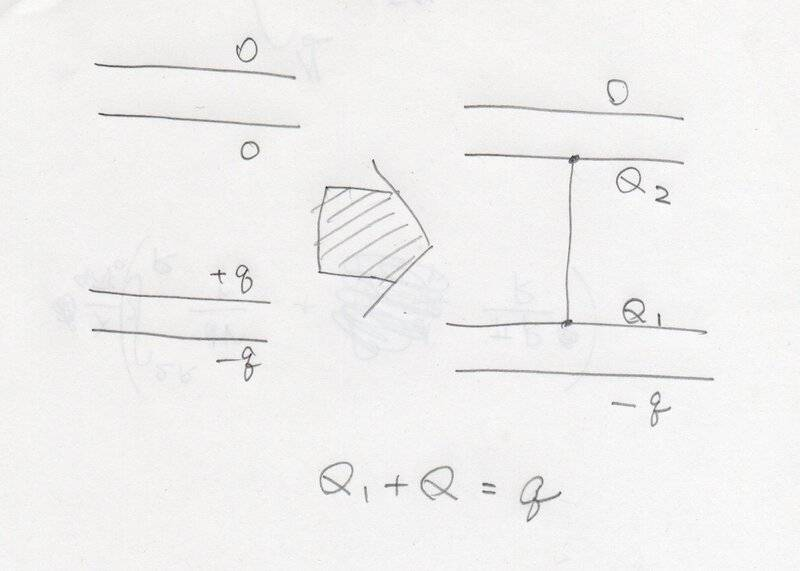# Electrostatic Influence and Series-Connected Capacitors

• I
• reterty
In summary, the assumption that the absolute values of the charges on the plates in a series circuit are equal is a basic assumption of circuit theory.f

#### reterty

As you know, "in the classics" the charges of the capacitor plates are equal in absolute value and opposite in sign. However, let us consider a series connection of capacitors. In this case, a charge of the opposite sign is induced on every second plate of each capacitor due to electrostatic induction. But how can we rigorously prove the strict equality of the absolute values of these charges to the charges of the first plates?

Do you mean configuration as attached?No, we consider the case of capacitors in series connected with battery. The first plate of the first capacitor has charge q, whereas the second plate of the last capacitor has charge -q. Intermediate plates are charged through influence and it is necessary to prove that their absolute values are also equal to q

This is one of the basic assumptions of circuit theory, that the current in a series branch is everywhere the same:

$$I(t)=\frac{dQ_1}{dt}=\frac{dQ_2}{dt}=...=\frac{dQ_n}{dt}$$ from which equality by integration you can deduce that all the charges differ by a constant, this constant being the charge each plate has at the time t=0. If all the capacitors are uncharged at t=0, then their charges are equal at any time t.

The above assumption holds only if the wavelength of the current in the circuit is big in comparison with the physical length of the series branch.

•Klystron, DaveE and vanhees71
Intermediate plates are charged through influence and it is necessary to prove that their absolute values are also equal to q
I think it is zero because no charge can enter into or go out from the intermediate part ,i.e. the second plate charged -q, the third plate charged q and the wire between non charged.

Here's another proof:
Say you have 4 unit-area plates stacked close together vertically. 4 plates, numbered 1 to 4, left to right. 3 gaps.

Number the surfaces 1 thru 8 left to right.

Put charge Q on plate 1 and -Q on plate 4. Assume the center two plates have zero charge.

Fact: the charges on each facing pair of surfaces (e.g. #2 & #3 or #4 & #5) must be equal and opposite, otherwise the divergence in the E field is non-zero which violates Maxwell.

Thus,
## \sigma_2 = \sigma_4 = \sigma_6 = -\sigma_3 =- \sigma_5 = -\sigma_7 ##.

But also, ## \sigma_1 + \sigma_2 = Q ## and
## \sigma_7 + \sigma_8 = -\sigma_2 + \sigma_8 = -Q ##.

Now put a + test charge inside plate 1. This charge will see a rightward force due to ## \sigma_1 ## and a leftward force force due to ## \sigma_8 ##. Note that ## \sigma_2 ## thru ## \sigma_7 ## forces cancel each other out.

That leaves us with
## \sigma_1 + \sigma_2 = Q ##
## \sigma_8 - \sigma_2 = -Q ##
and since the net force on the test charge must = 0,
## \sigma_1 - \sigma_8 = 0 ##.

OK look at these equations. They have no solution!
So the assumed charge distribution is impossible.

Matter of fact, no matter what charge you put on each plate, no matter how many plates, the outside 2 surfaces must have the same charge including sign!

Last edited: